login  home  contents  what's new  discussion  bug reports help  links  subscribe  changes  refresh  edit
Author
M.G. Richardson
Date Created
1996 Jan. 23
Related Constructors
Fraction
Source repository URL
http://wiki.fricas.org/axiom--test--1/src/algebra/FfracAs
N.B.
ndftip.as inlines this, must be recompiled if this is.

## Description:

This type represents formal fractions - that is, pairs displayed as fractions with no simplification.

If the elements of the pair have a type X which is an integral domain, a FFRAC X can be coerced to a FRAC X, provided that this is a valid type. A FRAC X can always be coerced to a FFRAC X. If the type of the elements is a Field, a FFRAC X can be coerced to X.

Formal fractions are used to return results from numerical methods which determine numerator and denominator separately, to enable users to inspect these components and recognise, for example, ratios of very small numbers as potentially indeterminate.

fricas
(1) -> <aldor>
#include "fricas.as"
FFRAC ==> FormalFraction ;
OF    ==> OutputForm ;
SC    ==> SetCategory ;
FRAC  ==> Fraction ;
ID    ==> IntegralDomain ;
FormalFraction(X : SC) : SC with {
-- Could generalise further to allow numerator and denominator to be of
-- different types, X and Y, both SCs.  "Left as an exercise."
/ : (X,X) -> % ;
++ / forms the formal quotient of two items.
numer : % -> X ;
++ numer returns the numerator of a FormalFraction.
denom : % -> X ;
++ denom returns the denominator of a FormalFraction.
if X has ID then {
coerce : % -> FRAC(X pretend ID) ;
++ coerce x converts a FormalFraction over an IntegralDomain to a
++ Fraction over that IntegralDomain.
coerce : FRAC(X pretend ID) -> % ;
++ coerce converts a Fraction to a FormalFraction.
}
if X has Field then coerce : % -> (X pretend Field) ;
import from Record(num : X, den : X) ;
Rep == Record(num : X, den : X) ; -- representation
((x : %) = (y : %)) : Boolean ==
((rep(x).num = rep(y).num) and (rep(x).den = rep(y).den)) ;
((n : X)/(d : X)) : % == per(record(n,d)) ;
coerce(r : %) : OF == (rep(r).num :: OF) / (rep(r).den :: OF) ;
numer(r : %) : X == rep(r).num ;
denom(r : %) : X == rep(r).den ;
if X has ID then {
coerce(r : %) : FRAC(X pretend ID)
== ((rep(r).num)/(rep(r).den)) @ (FRAC(X pretend ID)) ;
coerce(x : FRAC(X pretend ID)) : % == x pretend % ;
}
if X has Field then coerce(r : %) : (X pretend Field)
== ((rep(r).num)/(rep(r).den)) $(X pretend Field) ; }</aldor> fricas Compiling FriCAS source code from file /var/lib/zope2.10/instance/axiom-wiki/var/LatexWiki/5159521550557659159-25px001.as using Aldor compiler and options -O -Fasy -Fao -Flsp -lfricas -Mno-ALDOR_W_WillObsolete -DFriCAS -Y$FRICAS/algebra -I $FRICAS/algebra Use the system command )set compiler args to change these options. fricas Compiling Lisp source code from file ./5159521550557659159-25px001.lsp Issuing )library command for 5159521550557659159-25px001 fricas Reading /var/aw/var/LatexWiki/5159521550557659159-25px001.asy FormalFraction is now explicitly exposed in frame initial FormalFraction will be automatically loaded when needed from /var/aw/var/LatexWiki/5159521550557659159-25px001 fricas f1 : FormalFraction Integer Type: Void fricas f1 := 6/3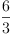(1) Type: FormalFraction(Integer) fricas -- 6 -- - -- 3 f2 := (3.6/2.4)$FormalFraction Float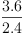(2)
Type: FormalFraction(Float)
fricas
--       3.6
--       ---
--       2.4
numer f1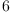(3)
Type: PositiveInteger?
fricas
--       6
denom f2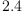(4)
Type: Float
fricas
--       2.4
f1 :: FRAC INT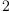(5)
Type: Fraction(Integer)
fricas
--       2
% :: FormalFraction Integer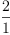(6)
Type: FormalFraction(Integer)
fricas
--       2
--       -
--       1
f2 :: Float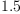(7)
Type: Float

On Sun, 01 Apr 2007 22:20:55 -0700 Tim Daly wrote:

I've attached the ffrac.spad domain. It handles "formal fractions" which do not evaluate the numerator or the denominator. Thus the formal fraction is just a data structure except that there are natural coercions to the algebra and back where they make sense.

This is related to the discussion of symbolic manipulation versus algebraic manipulation.

In particular, there are two books by Joel Cohen called "Computer Algebra and Symbolic Computation". I've had a discussion with Joel about his work (which he has agreed to allow Axiom to use at will). He defines MPL, a mathematical programming language, that basically does expression-tree manipulations.

I'd suggested that we could create a "Cohen Algebra" category and domain set that would follow his MPL style quite closely. The main thing would be to choose a common representatin (Record or List) for all elements of the domain so they compose properly.

The big difference in an axiom implementation would be to have coercions (or explicit import/export) from the Cohen domains to algebraic domains and back where they make sense.

This would satisfy the apparent end-user need to manipulate the expression trees.

This fits in layer 10:

)abbrev domain FFRAC FormalFraction
+++ Author: M.G. Richardson, Timothy Daly (spad rewrite)
+++ Date Created: 1996 Jan. 23
+++ Date Last Updated: 30 March 2007
+++ Basic Functions:
+++ Related Constructors: Fraction
+++ Also See:
+++ AMS Classifications:
+++ Keywords:
+++ References:
+++ Description:
+++ This type represents formal fractions - that is, pairs displayed as
+++ fractions with no simplification.
+++
+++ If the elements of the pair have a type X which is an integral
+++ domain, a FFRAC X can be coerced to a FRAC X, provided that this
+++ is a valid type.  A FRAC X can always be coerced to a FFRAC X.
+++ If the type of the elements is a Field, a FFRAC X can be coerced
+++ to X.
+++
+++ Formal fractions are used to return results from numerical methods
+++ which determine numerator and denominator separately, to enable
+++ users to inspect these components and recognise, for example,
+++ ratios of very small numbers as potentially indeterminate.
FormalFraction(X : SetCategory) : SetCategory with
_/: (X,X) -> %
++ x / y is the formal fraction of the input arguments
numer : % -> X
++ numer(x) is the numerator of the formal fraction
denom : % -> X
++ denom(x) is the denominator of the formal fraction
if X has IntegralDomain then
coerce : % -> Fraction(X)
++ coerce(x) from here to Fraction(there)
coerce : Fraction(X) -> %
++ coerce(x) from Fraction(there) to here
if X has Field then
coerce : % -> X
++ coerce from here to Field(there)
import Record(num : X, den : X)
Rep := Record(num : X, den : X)  -- representation
-- (a::Symbol / b::Symbol)$FFRAC(Symbol) -- c:Symbol:=d -- e:Symbol:=f -- (c/e)$FFRAC(Symbol)
(n:X / d:X):% == [n,d]
-- g:=(c/e)$FFRAC(Symobl) -- h:=(c/e)$FFRAC(Symbol)
-- (g=h)::Boolean
-- i:=(a::Symbol / b::Symbol)$FFRAC(Symbol) -- j:=(a::Symbol / b::Symbol)$FFRAC(Symbol)
-- (i=j)::Boolean
_=(x:%, y:%):Boolean == (x.num = y.num) and (x.den = y.den)
-- numer g
-- numer i
numer(r:%):X == r.num
--denom g
--denom i
denom(r:%):X == r.den
coerce(r:%):OutputForm == (r.num :: OutputForm) / (r.den :: OutputForm)
-- COMPLEX(INT) has FIELD
-- COMPLEX(INT) has IntegralDomain
-- p:COMPLEX(INT):=2
-- p::FRAC(COMPLEX(INT))::FFRAC(COMPLEX(INT))
if X has IntegralDomain then
coerce(r : %) : Fraction(X)
==  (r.num / r.den) @ Fraction(X)
coerce(x : Fraction(X)) : % == x pretend %
-- FRAC(POLY(INT)) has FIELD
-- m:=(x^2)@FRAC(POLY(INT))
-- m::FFRAC(POLY(INT))
if X has Field then
-- m:=(2/3)\$FFRAC(INT)
-- m::COMPLEX(FRAC(INT))
coerce(r : %) : X
== (r.num / r.den) @ X
   Compiling FriCAS source code from file
using old system compiler.
FFRAC abbreviates domain FormalFraction
------------------------------------------------------------------------
initializing NRLIB FFRAC for FormalFraction
compiling into NRLIB FFRAC
importing Record(num: X,den: X)
compiling exported / : (X,X) -> %
FFRAC;/;2X%;1 is replaced by CONS
Time: 0.00 SEC.
compiling exported = : (%,%) -> Boolean
Time: 0 SEC.
compiling exported numer : % -> X
FFRAC;numer;%X;3 is replaced by QCAR
Time: 0 SEC.
compiling exported denom : % -> X
FFRAC;denom;%X;4 is replaced by QCDR
Time: 0 SEC.
compiling exported coerce : % -> OutputForm
Time: 0 SEC.
****** Domain: X already in scope
augmenting X: (IntegralDomain)
compiling exported coerce : % -> Fraction X
Time: 0.01 SEC.
compiling exported coerce : Fraction X -> %
FFRAC;coerce;F%;7 is replaced by x
Time: 0 SEC.
****** Domain: X already in scope
augmenting X: (Field)
compiling exported coerce : % -> X
Time: 0.00 SEC.
****** Domain: X already in scope
augmenting X: (Field)
****** Domain: X already in scope
augmenting X: (IntegralDomain)
(time taken in buildFunctor:  0)
;;;     ***       |FormalFraction| REDEFINED
;;;     ***       |FormalFraction| REDEFINED
Time: 0 SEC.
Warnings:
 =:  num has no value
 =:  den has no value
 numer:  num has no value
 denom:  den has no value
 coerce:  num has no value
 coerce:  den has no value
Cumulative Statistics for Constructor FormalFraction
Time: 0.01 seconds
finalizing NRLIB FFRAC
Processing FormalFraction for Browser database:
--------constructor---------
--------(/ (% X X))---------
--------(numer (X %))---------
--------(denom (X %))---------
--------(coerce ((Fraction X) %))---------
--------(coerce (% (Fraction X)))---------
--------(coerce (X %))---------
; compiling file "/var/aw/var/LatexWiki/FFRAC.NRLIB/FFRAC.lsp" (written 18 SEP 2023 02:00:25 PM):
; wrote /var/aw/var/LatexWiki/FFRAC.NRLIB/FFRAC.fasl
; compilation finished in 0:00:00.008
------------------------------------------------------------------------
FormalFraction is already explicitly exposed in frame initial
FormalFraction will be automatically loaded when needed from
/var/aw/var/LatexWiki/FFRAC.NRLIB/FFRAC

 Subject:   Be Bold !! ( 14 subscribers )